## Using gnuplot to plot 3D graphs…

…with a little help from awk…

For instance the following awk took the list of the 1st 1000 prime numbers and added a column for the difference between each of the prime numbers:

“awk ‘{ p = \$1; getline; p = \$1 – p; print NR” “p” “\$1 }’ plist.txt > p8.txt”

Here’s the gnuplot file:

set dgrid3d 20,20
set hidden3d
set ticslevel 0
set term png
set out “p17.png”
splot “p8.txt” using 1:2:3 title “prime” with lines

And here’s the graph it created: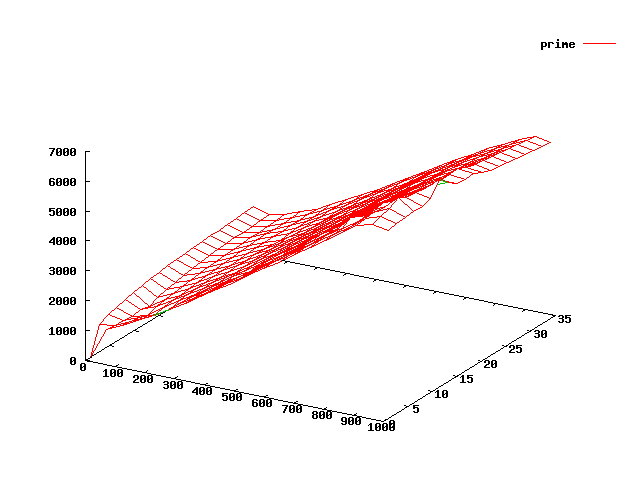1st 1000 prime numbers 3D graph -plotted against the difference between each of the prime numbers.

Here are some more graphs – again using the 1st 1000 prime numbers for data but for each one I’ve created another column of data based upon some manipulation of the primes: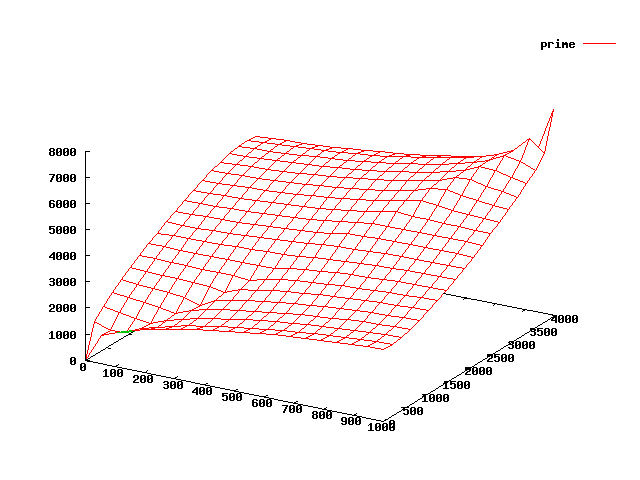3D plot of 1st 1000 prime numbers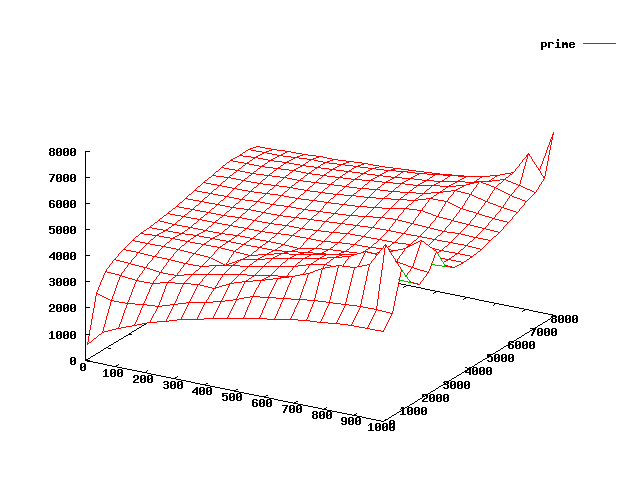3D graph plot of the 1st 1000 prime numbers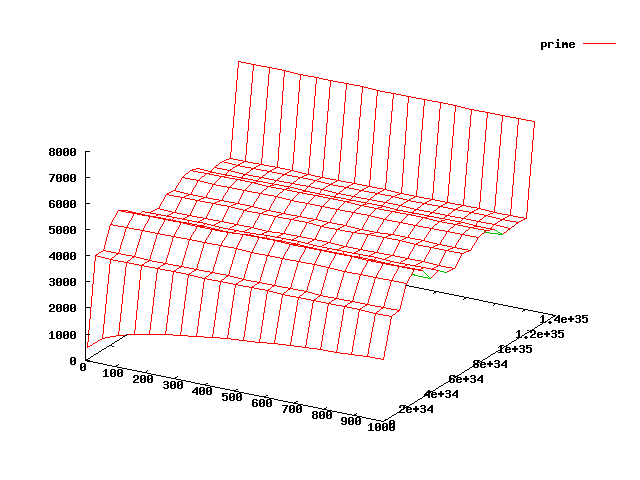3D plot of the 1st 1000 prime numbers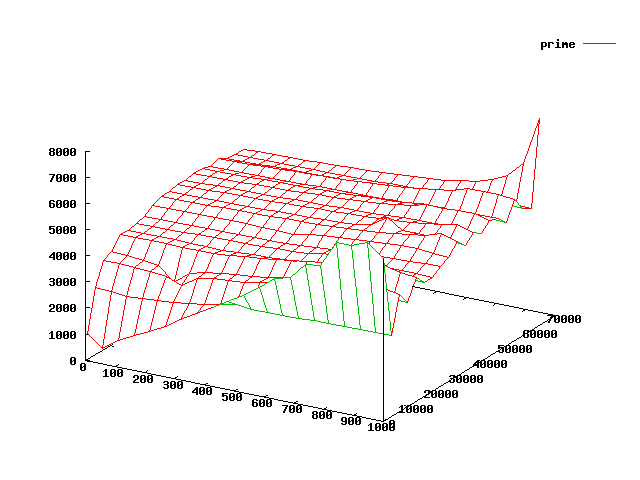3D gnuplot graph of the 1st 1000 prime numbers

## related articles

Comments are closed.